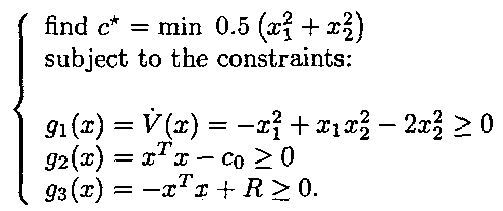# Help me to write a right cvx code

Hello
I want to find the ROA(Region of attraction) of a non-autonomous nonlinear system.
xdot=f(x(t))
x1dot=x2+(0.5+0.5x1)u
x2dot=x1+(0.5-4(1-2
x2)u

this doesn’t cover calculating the ROA of such systems
what should I do?

I’ve read some cvx codes but I want to sure my cvx code is right.

The objective function is:
where x=[x1;x2];

maximize(alfa)
s.t:
|K*x|<=2
x’Px<=alfa

my cvx code:

A=[-1.059 -0.0590; -0.0590 -1.059];
K=[2.1180 2.1180];
P=[0.473613603238296 -0.0263864036958539;-0.0263864036958539 0.473613603238295];

cvx_begin
variable x(2)
variable alfa(1,1) symmetric
maximize(alfa)
subject to
x’Px<=alfa
abs(K*x)<=2
cvx_end

It sounds ike your question has to do with the mathematics of determining ROA, and is not a question about how to implement a mathematical optimization or feasibility problem in CVX. Therefore, you are advised to seek a more suitable forum for assistance. If after doing so, you have a convex optimization (or feasibility) problem which you have difficulty implementing in CVX, then you can ask for such specific assistance on this forum at that time.

1 Likewhere c0=2
R=15
How can I formulate it in cvx!
I’ve tried many times but I couldn’t write it.
I have downloaded many papers to estimate the ROA… I think this is my last hope.

Regards

Neither of your first two constraints are convex. (For the first constraint, the Hessian of the left-hand side is not negative semi-definite, as it needs to be for a >= constraint to be convex, for any x_1 > 1 and x_2 = 1, as an example). Therefore, this problem can not be formulated with CVX.

You need to use another optimization tool, of which there are many which can handle this problem.

Note:the categorization of your question as being Nonconvex is based on your 2nd post of the thread, not necessarily to your original post.

1 Like

could you please recommend me a suitable optimization tool to solve my problem?
believe me my time is limited! in the mid of solving a model predictive control problem I have to estimate the ROA…If you need the global optimum, try BARON or BMIBNB (under YALMIP). Otherwise, try a general purpose nonlinear optimizer, such as FMINCON, IPOPT, KNITRO, or SNOPT, among many others.

Anyhow, I can spare you the effort for the problem and parameter values you provided - it is infeasible.

1 Like